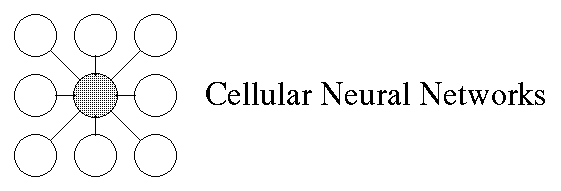### Internet related sites

Cellular Neural Networks (CNN) is a massive parallel computing paradigm defined in discrete N-dimensional spaces. Following the Chua-Yang definition:
• A CNN is an N-dimensional regular array of elements ( cells);
• The cell grid can be for example a planar array with rectangular, triangular or hexagonal geometry, a 2-D or 3-D torus, a 3-D finite array, or a 3-D sequence of 2-D arrays ( layers );
• Cells are multiple input-single output processors, all described by one or just some few parametric functionals ;
• A cell is characterized by an internal state variable , sometimes not directly observable from outside the cell itself;
• More than one connection network can be present, with different neighborhood sizes;
• A CNN dynamical system can operate both in continuous (CT-CNN) or discrete time (DT-CNN);
• CNN data and parameters are typically continuous values;
• CNN operate typically with more than one iteration, i.e. they are recurrent networks.
CNN main characteristic is the locality of the connections between the units: in fact the main difference between CNN and other Neural Networks paradigms is the fact that informations are directly exchanged just between neighboring units. Of course this characteristic allows also to obtain global processing. Communications between non directly ( remote ) connected units are obtained passing through other units. It is possible to consider the CNN paradigm as an evolution of Cellular Automata paradigm. Moreover it has been demonstrated that CNN paradigm is universal, being equivalent to the Turing Machine. A mathematical formal description of the discrete time case is contained in the following equations.

x(t+1) = g(x(t)) + I(t) + SUM( A(yk(t),PA(j)) ) + SUM( B(uk(t),PB(j)) )

y(t) = f (x(t))

where x is the internal state of a cell, y its output, u its external input and I a local value called bias . A and B are two generic parametric functionals, PA(j) and PB(j) are the parameters arrays (typically the inter-cell connection weights). The neighbor yk and uk values are collected from the cells present in the two neighborhood Nr , for the feedback functional A , and Ns , for the control functional B . The two neighborhoods are potentially different. The functionals A and B are also called templates . The instantaneous local feedback function g express the possibility of an immediate feedback effect. This function is typically not used. f is the function that gives cell output from the internal state. Generally is used the chessboard distance convention, expressed by the equation

d(i,j) = max(|x(i) - x(j)|,|y(i)-y(j)|)

In most of cases the system is non-markovian , i.e. the future internal state depends also from the past history of the system. In the special case of time-variant CNN all the above functions, neighborhoods and parameters can be also function of time. In figure is depicted a block-scheme of a generical CNN iteration.### Internet resources:

#### Cellular Neural Networks Ftp Site at University of Trento, Italy.

• Ftp site manager:
Roberto Battiti
battiti@itnvax.science.unitn.it (130.186.34.2)

### Internet Pointers (Papers):

Back to Parma Neural Networks Page
Back to Parma Cellular Automata Page
Back to Parma Parallel and Distributed Processing Page
Page Manager:

Giulio Destri (destri@ce.unipr.it)